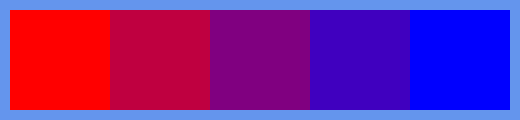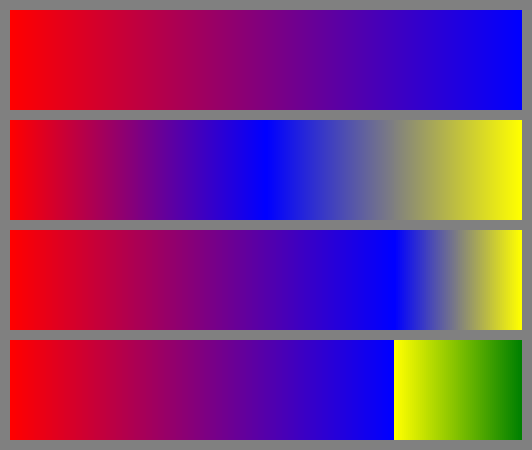# Colour interpolation in generativepy

By Martin McBride, 2021-11-16
Tags: generativepy tutorial colour colormap lerp
Categories: generativepy generativepy tutorialColour interpolation allows you to create a new colour that is partway between two existing colours. It can be thought of as mixing the two colours.

The technique uses linear interpolation and is sometimes called lerping as a contraction of that term. generativepy provides two ways of doing this:

• The lerp method of the Color class provides simple lerping for two colours.
• The make_colormap function creates a colour map, containing a list of varying colours.

## lerp method

Here is a simple example of how to use the lerp method:

red = Color('red')
blue = Color('blue')
mix = red.lerp(blue, 0.25)


We first create two Color objects, red and blue.

When we call the lerp method of the red object, we pass in a second colour blue and a factor of 0.25.

This creates a third Color object, mix that consists of 75% red, 25% blue.

Here is a longer example that creates a set of colour squares with colour that mix varying degrees of red and blue:

from generativepy.drawing import make_image, setup
from generativepy.color import Color
from generativepy.geometry import Rectangle

def draw_lerp(ctx, pixel_width, pixel_height, frame_no, frame_count):
setup(ctx, pixel_width, pixel_height, background=Color('cornflowerblue'))

color1 = Color('red')
color2 = Color('blue')

pos = [10, 10]
w = 100
h = 100

Rectangle(ctx).of_corner_size(pos, w, h).fill(color1.lerp(color2, 0))
pos += w
Rectangle(ctx).of_corner_size(pos, w, h).fill(color1.lerp(color2, 0.25))
pos += w
Rectangle(ctx).of_corner_size(pos, w, h).fill(color1.lerp(color2, 0.5))
pos += w
Rectangle(ctx).of_corner_size(pos, w, h).fill(color1.lerp(color2, 0.75))
pos += w
Rectangle(ctx).of_corner_size(pos, w, h).fill(color1.lerp(color2, 1))
pos += w

make_image("colour-lerp.png", draw_lerp, 520, 120)


This code is available on github in tutorial/colour/colour_lerp.py.

Here is the image created by the code above. It contains colours from red through magenta to blue, in 5 stages:## make_colormap function

A colour map is a list of colours. You can use it to map integer values onto colours. This is very useful, for example, if you have created a fractal image that contains integer values across a particular range. You can use the colour map to convert those integers to colours.

A colour map can contain two or more different colours and allows for smooth variation between colours. You can also introduce sharp colour changes.

Here is the simplest type of colour map:

colormap = make_colormap(256, [Color('red'), Color('blue')])


The size of the map is 256. The start and end colours (in the array) are red and blue. The colormap returned is a Python list of Color objects. Here are some example entries:

• colormap is pure red.
• colormap is mostly red with a tiny bit of blue.
• colormap is magenta (half red, half blue).
• colormap is mostly blue with a tiny bit of red.
• colormap is pure blue.

You can make the colour map any size you wish. So for example, if you have a fractal image with values in the range 0 to 599, you can make a map of size 600.

In the example code below, we create that red/blue map above and use the colours to create 256 narrow rectangles, side by side. This gives the illusion of a continuous variation of colour from red to blue. The example code repeats this with 3 other color maps:

from generativepy.drawing import make_image, setup
from generativepy.color import Color, make_colormap
from generativepy.geometry import Rectangle

def draw_map(ctx, pixel_width, pixel_height, frame_no, frame_count):
setup(ctx, pixel_width, pixel_height, background=Color(0.5))

w = 2
h = 100

pos = [10, 10]
colormap = make_colormap(256, [Color('red'), Color('blue')])
for i in range(256):
Rectangle(ctx).of_corner_size(pos, w, h).fill(colormap[i])
pos += 2

pos = [10, 120]
colormap = make_colormap(256, [Color('red'), Color('blue'), Color('yellow')])
for i in range(256):
Rectangle(ctx).of_corner_size(pos, w, h).fill(colormap[i])
pos += 2

pos = [10, 230]
colormap = make_colormap(256, [Color('red'), Color('blue'), Color('yellow')], [3, 1])
for i in range(256):
Rectangle(ctx).of_corner_size(pos, w, h).fill(colormap[i])
pos += 2

pos = [10, 340]
colormap = make_colormap(256, [Color('red'), Color('blue'), Color('yellow'), Color('green')], [3, 0, 1])
for i in range(256):
Rectangle(ctx).of_corner_size(pos, w, h).fill(colormap[i])
pos += 2

make_image("colour-map.png", draw_map, 532, 450)


The second colour map is:

colormap = make_colormap(256, [Color('red'), Color('blue'), Color('yellow')])


This creates a map that goes from red to blue to yellow. There are 2 colour transitions, and each is the same size. So:

• colormap is pure red.
• colormap is pure blue.
• colormap is pure yellow.

With smooth transitions in between.

The third colour map is:

colormap = make_colormap(256, [Color('red'), Color('blue'), Color('yellow')], [3, 1])


This is similar to before, but we have a bands value of [3, 1]. This gives the relative sizes of the bands. It specifies that the transition from red to blue is 3 times wider than the transition from blue to yellow. So:

• colormap is pure red.
• colormap is pure blue. This is 75% of the way along the map.
• colormap is pure yellow.

The fourth and final colour map is:

colormap = make_colormap(256, [Color('red'), Color('blue'), Color('yellow'), Color('green')], [3, 0, 1])


This time we have 4 colours, so we need 3 band values. In this case, they are [3, 0, 1]. The zero band size specifies a sharp transition so:

• colormap to colormap varies smoothly from red to blue.
• colormap to colormap varies smoothly from yellow to green.

Between points 191 and 192 there is a step-change from blue to yellow, with no intermediate colours.

This code is available on github in tutorial/colour/colour_map.py.

Here is the image created by the code above. It shows all 4 colour maps: